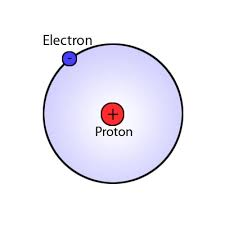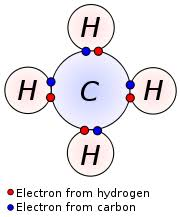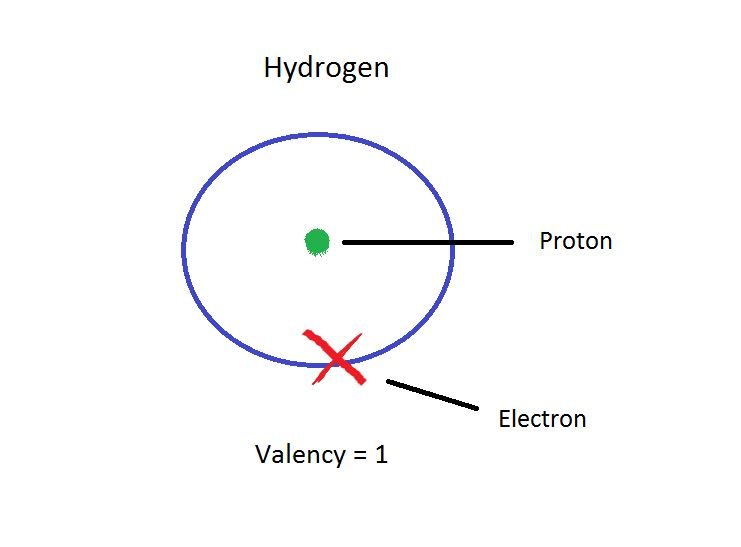# What is The Total Number of Valence Electrons in a Hydrogen Atom

Today we shall take up the topic What is the Valency of Hydrogen. Valency is an important concept in chemistry and has been used to formulate many theories in chemistry and science in general. It can be found by looking at the group number of an element in a periodic table.###Since Hydrogen has only one electron in its shell, its valency is 1. It can also be found out by looking at the periodic table since Hydrogen belongs to group 1. So to achieve stability it needs to share a bond with only one electron of another atom.

Hence one hydrogen atom can form bonds with electrons of many atoms simultaneously.

For example, four Hydrogen atoms can form a bond with a carbon atom, to form the compound methane (CH4).

### How Do You Find The Valence Electrons For Hydrogen

Since Hydrogen belongs to the first group of elements its electronic configuration is 1. Hence it belongs to the first group of the periodic table. And if the electronic configuration of any element is less than or equal to four, its valency can be determined by the group it belongs to in the periodic table.### What is The Total Number of Valence Electrons in a Hydrogen Atom

A Hydrogen atom has only one electron in its outer most shell. Hence it requires to combine with one more electron of an atom to achieve stability. So its number of valence electrons is 1.